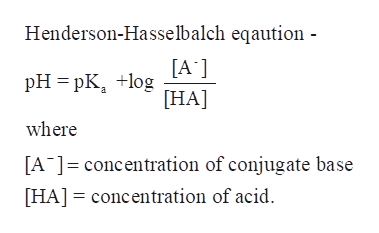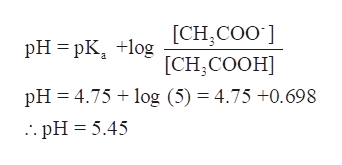# If the acetate to acetic acid ratio of a solution is five, what is its pH? The pKa of acetic acid is 4.75.

Question
20 views

If the acetate to acetic acid ratio of a solution is five, what is its pH? The pKa of acetic acid is 4.75.

check_circle

Step 1

We have to determine the pH of a solution which has both acetic acid and acetate ion and the acetate to acetic acid ratio of a solution is five.

The pKa of acetic acid is 4.75.

Step 2

A buffer solution has a mixture of a weak acid and its conjugate base or vice-versa. Thus, the given solution is buffer solution as acetic acid is a weak acid and acetate ion is its conjugate base.

The Henderson-Hasselbalch equation is used to determine the pH of a buffer solutionhelp_outlineImage TranscriptioncloseHenderson-Hasselbalch eqaution - pHpK [A'] [HA where [A concentration of conjugate base [HA] concentration of acid fullscreen
Step 3

Now calculate the pH of the given solution using the given data-

...help_outlineImage TranscriptionclosepH pK gCH,COO] [CH,CООН] pH 4.75 log (5) = 4.75 +0.698 . pH 5.45 fullscreen

### Want to see the full answer?

See Solution

#### Want to see this answer and more?

Solutions are written by subject experts who are available 24/7. Questions are typically answered within 1 hour.*

See Solution
*Response times may vary by subject and question.
Tagged in

### General Chemistry